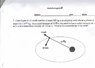# Elliptical orbit question

• superaznnerd
If he had wanted to solve for "a", he would have needed to look up the first equation and substitute in "a" for "e". And if he had wanted to solve for "eccentricity", he would have needed to look up the second equation and substitute in "eccentricity" for "a".f

see attachment

## Homework Equations

2a (average distance of satellite from central body) = A (aphelium) + P (perihelium)
ME = KE + PE
ME = -GMm/24
KE = .5mv^2
PE = -GMm/r
ME orignall = ME final

## The Attempt at a Solution

I tried to put ME = KE + PE = constant.
I solved for theoretical A, and P, if the velocity was only transverse.
Then, I subtracted the theoretical P by the distance caused by the KE. I sitll did not get the answer htough

#### Attachments

•ellipt orbit.jpg
26.7 KB · Views: 522
Last edited:

Please list all of the formulas and steps you did. Not sure what you are defining as A and P.

anyone?

Given the components of the velocity, can you determine the angle at that point? What is the polar equation for an ellipse in terms of the eccentricity?

There is a formula for eccentricity in terms of energy and angular momentum. If you don't know it, then you will have to solve for it using the conservation of energy and the conservation of angular momentum.

I looked at the wiki page, and have no clue hwo to apply conservation of angular momentum. could someone please show me how to do it?

Calculate the angular momentum of the satellite using

$$\vec{L} = m\vec{r}\times \vec{v}$$

Don't even bother with the satellite mass. It's just going to divide out in the end. Just use specific energy and specific angular momentum.

I looked at the wiki page
Which wiki page? There are several of them.

and have no clue hwo to apply conservation of angular momentum. could someone please show me how to do it?
No, we can't show you how to do it. That would be cheating.

Can you look up the polar form of the equation for an ellipse, in terms of r, $\theta$, and the eccentricity? Can you find the angle, given the components of the velocity?

The polar form of an ellipse has two unknowns: 'a' and eccentricity. So it won't be enough to solve for the eccentricity alone.

I think the original poster has moved on by now.

The sad thing is that the word "eccentricity" is in big bold letters in the attachment in the original post. The OP claims to have "read the wiki page". Which one, I don't know. The wiki page on "Eccentricity" is a redirection page, and one of the redirects is to "Orbital eccentricity". The first equation specifies how to solve this exact problem in general; the third equation specializes this first equation to gravitation. In other words, exactly what the OP needed to use to solve this problem is right there, right up front, on "the wiki page".